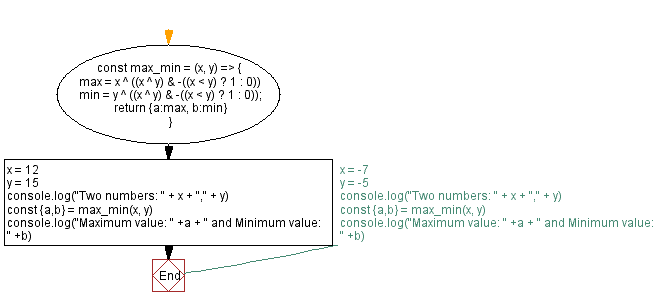# JavaScript - Maximum, minimum of two integers

## JavaScript Bit Manipulation: Exercise-15 with Solution

Write a JavaScript program to calculate the maximum or minimum of two integers.

Test Data:
(12, 15) -> 15, 12
(-7,-5) -> -5, -7

Sample Solution:

JavaScript Code:

`````` const max_min = (x, y) => {
max = x ^ ((x ^ y) & -((x < y) ? 1 : 0))
min = y ^ ((x ^ y) & -((x < y) ? 1 : 0));
return {a:max, b:min}
}
x = 12
y = 15
console.log("Two numbers: " + x + "," + y)
const {a,b} = max_min(x, y)
console.log("Maximum value: " +a + " and Minimum value: " +b)
// x = -7
// y = -5
// console.log("Two numbers: " + x + "," + y)
// const {a,b} = max_min(x, y)
// console.log("Maximum value: " +a + " and Minimum value: " +b)
```
```

Sample Output:

```Two numbers: 12,15
Maximum value: 15 and Minimum value: 12
```

Flowchart:Live Demo:

See the Pen javascript-bit-manipulation-exercise-15 by w3resource (@w3resource) on CodePen.

* To run the code mouse over on Result panel and click on 'RERUN' button.*

Improve this sample solution and post your code through Disqus

What is the difficulty level of this exercise?

Test your Programming skills with w3resource's quiz.

﻿